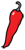The Grand Locus / Life for statistical sciences

## Poetry and optimalityClaude Shannon was the hell of a scientist. His work in the field of information theory, (and in particular his famous noisy channel coding theorem) shaped the modern technological landscape, but also gave profound insight in the theory of probabilities.

In my previous post on statistical independence, I argued that causality is not a statistical concept, because all that matters to statistics is the sampling of events, which may not reflect their occurrence. On the other hand, the concept of information fits gracefully in the general framework of Bayesian probability and gives a key interpretation of statistical independence.

Shannon defines the information of an event with probability $Prob(A)$ as $-\log P(A)$. For years, this definition baffled me for its simplicity and its abstruseness. Yet it is actually intuitive. Let us call $\Omega$ the system under study and $\omega$ its state. You can think of $\Omega$ as a set of possible messages and of $\omega$ as the true message transmitted over a channel, or (if you are Bayesian) of $\Omega$ as a parameter set and $\omega$ as the true value of the parameter. We have total information about the system if we know $\omega$. If instead, all we know is that $\omega$ is in the set $A$, we have imperfect information. It seems natural that we get more information if the set $A$ is small, or more precisely if it has a small probability. As you can see, the information term $-\log P(A)$ is 0 for $\Omega$ and infinitely high as $Prob(A)$ tends to 0.

With the information score at hand, we can compute the expected information of a random experiment. If the only two outcomes are that we observe $A$ or not, the expected information of such an experiment is

$$-P(A) \log P(A) - (1-P(A)) \log(1-P(A)).$$

This shows that even before sampling, not all random experiments have the same information potential. By differentiating the equation above, it appears that the experiment with the highest expected information is such that $P(A) = 1/2$, i.e. that both outcomes are equally likely. In other cases, one of the outcomes is more informative, but because it is less likely, it contributes less to the expected information. So if you have the choice between two Bernouilli trials, you should choose the one for which the probability is closer to 1/2 because you are more likely to learn more about the state of the system.

Now if we can observe two events of fixed probabilities, say $P(A)$ and $P(B)$, we can ask what is the optimal overlap between them so as to maximize the expected information of the trial. Standard calculations with Lagrange multipliers show that the optimum is reached for $P(A \cap B) = P(A)P(B)$, that is, if $A$ and $B$ are independent.

This property extends to more than two events and to random variables. As a result, statistical independence can be understood as an optimality property. For a given set of random variables or random events, independence is the joint distribution that maximizes the expected information of a random experiment. Independence means optimal sampling. If sampling is in reality not independent, the information gained in the course of the experiment will be overestimated, explaining why this usually gives too optimistic confidence intervals, and very low p-values.

Finally, what is the link between poetry and optimality? Nothing, really. Except maybe that Claude Shannon considered himself a great poet. To the editor of the Scientific American he wrote

I am a better poet than scientist.

along with a poem of which I copied the first words below and that you can read in full here.

A Rubric on Rubik Cubics

Strange imports come from Hungary:
Count Dracula, and ZsaZsa G.,
Now Erno Rubik’s Magic Cube
For PhD or country rube.
This fiendish clever engineer
Entrapped the music of the sphere.
It’s sphere on sphere in all 3D—
A kinematic symphony!
Ta! Ra! Ra! Boom De Ay!
One thousand bucks a day.
That’s Rubik’s cubic pay.
...

Well Claude, keep the day job, as they say.